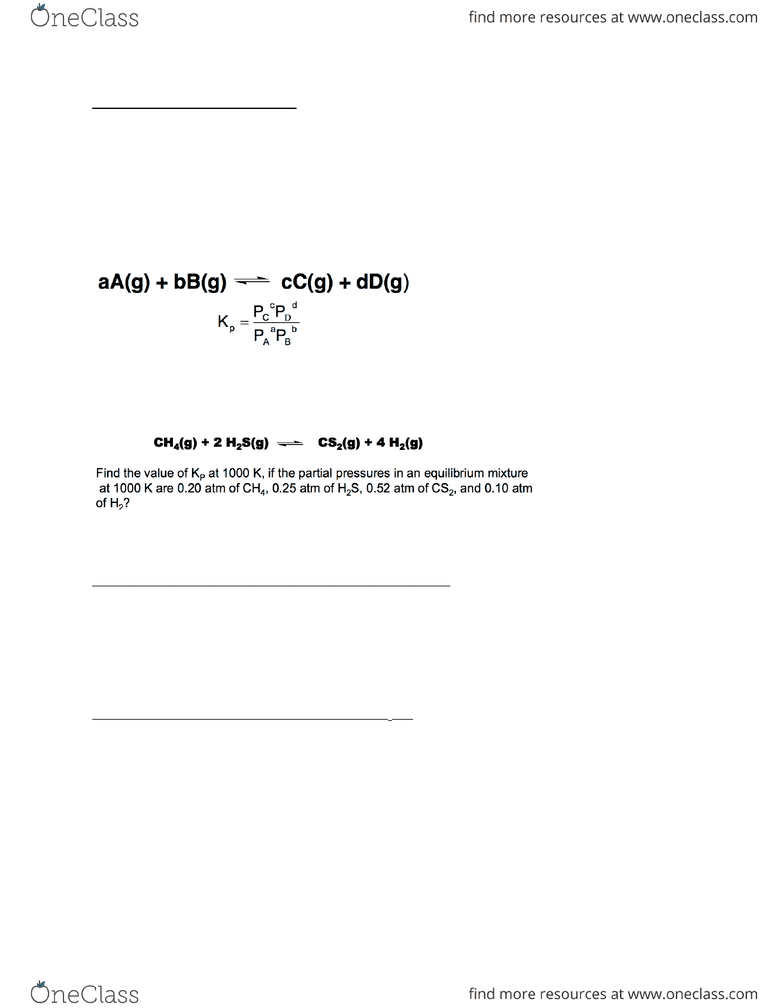Class Notes (1,100,000)
CA (620,000)
UTSG (50,000)
CHM (800)
CHM135H1 (400)
Lecture 14

# CHM135H1 Lecture Notes - Lecture 14: Partial Pressure, Bromine, Reaction Quotient

Department
Chemistry
Course Code
CHM135H1
Professor
Kris Quinlan
Lecture
14

This preview shows page 1. to view the full 4 pages of the document.Summary of the previous lecture
In reacon equilibrium, the rates of the forward and reverse reacons are equal
General form: aA + bB -> <- cC + dD
Kc = k1/k-1
Kc= [C]c[D]d/[A]a[B]b
oKc is dimensionless, concentraons are divided by 1 mol before this
No net changes on the macroscopic level and on the molecular level
Gases (Ideal Gases only)
relave to 1 atm*
Noce that you are dealing with Kp (and not Kc as they are not necessarily equal)
Paral pressure of each = [A]RT; [A] is the concentraon of the gas
Kp = Kc(RT)# of moles
oIf sum of moles is equal to 0, Kp = Kc
Start from most general equaon, Kp and plug in the coe7cients of each
Plug in the pressures (in atm, NOT mmHg – divide these by 1 atm to remove units)
Solve for the answer, Kp
Write equilibrium expressions for the following reacons:
3H2(g) + SO2(g)  H2S(g) + 2H2O(g)
Kp = PH2SPH2O2/PH23PSO2
2C2F5Cl(g) + 4O2(g)  Cl2(g) +4CO2(g) +5F2(g)
Soluons
All units are relave to 1M
Write the equilibrium-constant expression for Kc for
Ag+(aq) + 2NH3(aq)  Ag(NH3)2+(aq)
Kc = [Ag(NH3)24/[Ag+][NH3]2
Cd2+(aq) + 5Br-  CdBr42-(aq)
Kc = [CdBr42-]/[Cd2+][Br-]4
Heterogeneous Equilibria (mixture of states)
Approach: the concentraons of pure solvents/liquids are not included in the
equilibrium expression (when we plug in the numbers, instead of their concentraons,
we can use 1) – assumed that the concentraon does not change
###### You're Reading a Preview

Unlock to view full version